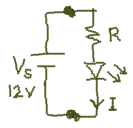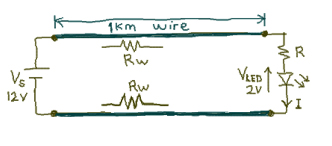# How to choose a suitable size of Cable & Wire

Cable is like a pipe. Charges is like the water. Current is like the water flowing rate through the pipe, volume of litres per sec. Voltage is like the water pressure from one point to another inside the pipe. Energy is like the water meter terminal located at the outlet of individual home, counting the accumulating charges that reaches our home. This is how we can have an alternative view on electricity. Given a fix water pressure.

Everybody is aware that thicker wire has less resistance. Have you ever wonder if more current can ever be resultant from the use of a thicker wire? Will it result in more current flow? In a typical power system setup, replacing with a thicker wire does not result in higher current consumption, but an increase in the current carrying capacity of the cable. Increasing the wire size does have a little effect to a whole circuit.

The follow article explains the implication of substituting a finer wire with a thicker wire and discuss the typical consideration in choosing a suitable wire size.

Schematic (1) illustrate a simple LED circuit assuming no cable or cable that have no resistance. The calculated values are design to drive the LED at 10mA.Schematic (1) I= 10mA R=1000Ω Vled= 2V Vs= 12V (constant voltage supply)

Schematic (2) illustrate the same LED circuit 1km away from power supply connected by a wire (cross sectional area of 1.1mm2). A wire of 1.1mm2, has a typical resistance of 15.2Ω/km at room temperature. Using a constant voltage supply, the current flowing through the wire can be computed to be,

I= (Vs – Vled)/(R+Rw+Rw), where Rw= 15.2Ω
= (12V-2V)/(1000Ω+15.2Ω+15.2Ω)
= 9.7mASchematic (2) I= 10mA R=1000Ω Vled= 2V Vs= 12V (constant voltage supply) Rw= 15.2Ω for 1km wire

By including a 1km long cable to the ideal system illustrated in schematic (1), it results in a slight current drop of 0.3mA.

Schematic (3) illustrate the same LED circuit setup as schematic (2) but the 1.1mm2 wire is swap with a 3.1mm2 wire. A wire of 3.1mm2, has a typical resistance of 5.47Ω/km at room temperature. Using a constant voltage supply, the current flowing through the wire can be computed to be

I= (Vs – Vled)/(R+Rw+Rw), where Rw= 5.47Ω for 1km
= (12V-2V)/(1000Ω+5.47Ω+5.47Ω)
= 9.89mASchematic (3) I= 10mA R=1000Ω Vled= 2V Vs= 12V (constant voltage supply) Rw= 5.47Ω for 1km wire

By increasing the wire size by 3 times, reducing the resistance by 3 times, there is only a slight increase in current flow from 9.7mA to 9.89mA. As the cable resistance is reduce, the current that flows through the cable will approach towards 10mA. A extreme situation where cable is lossless, current will be 10mA. This will be the ideal case illustrated in schematic (1).

Schematic (3), (2) and (1) have demonstrate that by increase the wire size (or reducing the wire resistance) by roughly 3 times, it does little effect to a properly design system. The current flowing through the wire has almost negligible changes. By reducing the cable resistance by 3 times, it will not increase the current flow by 3 times.

You may think that it does not sounds like the property of ohms law, but on careful analysis, it does no conflict to the electrical law. It is actually the fact that ohms law can be use for various type of design, meant for different application.

A proper design power system should be able to deliver all it power to the intended load. Therefore the resistance of the wire should be design to be a lot less than the resistance of the system. An ideal wire system will be 0Ω as illustrated in schematic (1). In conventional application, cable resistance of 0Ω is not possible. As long as the cable size selected do not affect the load much, it is consider good enough. Most practical design is approximated the ideal case in schematic (1), if the cable resistance is <0.1% of the load’s resistance. However take note that this guide is subjective and varies from system to system, depending on your application.

Schematic (4) illustrate a improper design with wire chosen to have close resistance to the load. For illustration purpose a 0.049mm2 wire is chosen. A typical 0.049mm2 wire has about 351Ω/km To maintain the same lighting strength of about 10mA, R has to be about 298Ω.

Such a design is very sensitive to the resistance of the cable, and can varies a lot when temperature changes. It will be consider a good design if it is meant temperature measuring application. Since the purpose of the cable is to deliver power, this type of design is considered as an improper design.

By changing the thin wire with a thicker wire of 0.096mm2 (178Ω/km) in the system, the current flowing through the system can be computed to be,

I= (Vs – Vled)/(R+Rw+Rw), where Rw= 178Ω for 1km
= (12V-2V)/(298Ω+178Ω+178Ω)
= 15.29mASchematic (4) I= 10mA R=298Ω Vled= 2V Vs= 12V (constant voltage supply) Rw= 178Ω for 1km wire

Note that in this design, the reduce in wire resistance by 50% (from 351Ω to 178Ω) has resulted in 50% increase in the current (from 10mA to 15.29mA) flowing through the circuits.

Personally, I would recommend having some safety buffer when choosing the right size of cable to use. I usually choose a cable size double the proper designed capacity. Two pillar is a lot safer than one. If you know that your load draws a maximum of 1A, use a cable that is thick enough to carry 2A. Any variation in temperature will not deviate much from the designed current capacity. It also helps to accumulate any accidental flow of current flowing through the wire, which will not burn the wire easily. The cut off of the current should be carried out by the fuse or MCB system. Therefore the rating of a fuse or MCB must always be smaller than that of a wire and the rest of the load. Imagine if a fuse has larger current capacity than the wire. It is a possible a situation where current is sufficient to heat up and start your wire burning without breaking the safety fuse. The wire could be break before your fuse is blown. This is one of the main causes for fire.

Examples illustrated are base on simple DC circuit model, with ideal voltage source, constant resistance, temperature, etc.

Related Products：